# How to Create Array Of Numbers Using Excel INDEX Function

In this article, we will learn How to Create list or array of numbers in Excel 2016.

At Instance, we need to get the list of numbers in order, which can be used in a formula for further calculation process. Then we try to do it manually, which results in multiple complications like errors or wrong formula.

How to solve the problem?

First we need to understand the logic behind this task. To create a list we need to know first number and last number. On the basis of the record, we will make a formula out of it. To solve this problem we will be using two excel functions.

Generic Formula:

= ROW ( INDIRECT ( start_ref & " : " & end_ref )

NOTE: This formula will return the list of numbers form num1 to num2. Use the Ctrl + Shift + Enter while using using the formula with other operational formulas.
Example:

Let's understand the formula with some data in excel.

First we will learn the easy way out to this task. Here the formula generates an array of numbers from 5 to 10.

Write the formula in the D2 cell.

Formula:

= ROW ( 5:10 )

5 : Start_num

10 : end_num

Explanation :

After the formula is used in the cell, the single output of the cell is shown as 5 in the gif below. But the array lays inside the cell. When I clicked the formula in the formula bar, and press F9 the cell shows me the array = {5 ; 6 ; 7 ; 8 ; 9 ; 10) , the resulting array.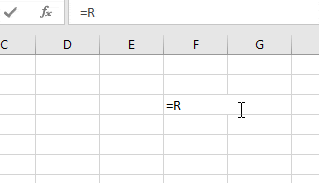The above gif explains the process to obtain an array { 5 ; 6 ; 7 ; 8 ; 9 ; 10}

Now we obtain when we take the numbers as provided as input and operations performed over the arrays.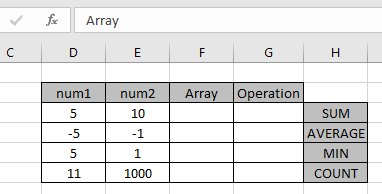Straight array

For the first array we will use the formula using the Generic formula above

Use the Formula:

= ROW ( INDIRECT ( D3 & ":" & E3 ) )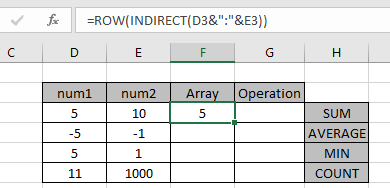Explanation :

After the formula is used in the cell, the single output of the cell is shown as 5 in the gif below. But the array lays inside the cell. When I clicked the formula in the formula bar, and press F9 the cell shows me the array = { 5 ; 6 ; 7 ; 8 ; 9 ; 10 } , the resulting array.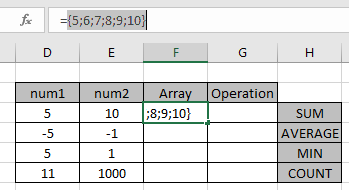After Using the F9 shortcut the array look like as shown in the image above.

Operation: Find the SUM of numbers from 5 to 10.
For this we will use the SUM function with array formula.
Use the formula in the G3 cell.

{ = SUM ( ROW ( INDIRECT ( D3 & ":" & E3 ) ) ) }

Explanation :
The above finds the SUM of the array = 5 + 6 + 7 + 8 + 9 + 10 = 45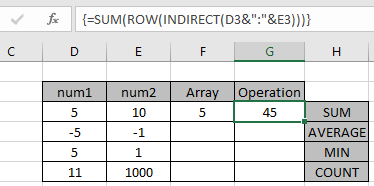The array formula works fine with the SUM function to return value as 45.

NOTE: This formula will return the list of numbers form num1 to num2. Use the Ctrl + Shift + Enter while using using the formula with other operational formulas. Curly braces is shown in the image above for the same.
Negative numbers : array

For the Second array we will use the below formula using the Generic formula explained above

Use the Formula:

= ROW ( INDIRECT ( 1 & ":" & 5) ) - 6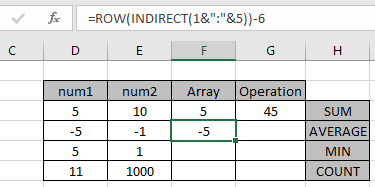Explanation :

After the formula is used in the cell, the single output of the cell is shown as -5 in the gif below. But the array lays inside the cell. When I clicked the formula in the formula bar, and press F9 the cell shows me the array = { -5 ; -4 ; -3 ; -2 ; -1 } , the resulting array.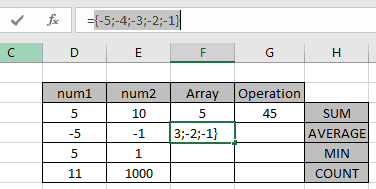After Using the F9 shortcut the array look like as shown in the image above.

Operation: Find the AVERAGE of numbers from -5 to -1.
For this we will use the AVERAGE function with array formula.
Use the formula in the G3 cell.

{ = AVERAGE ( ROW ( INDIRECT ( 1 & ":" & 5 ) ) - 6 ) }

Explanation :

The above finds the AVERAGE of the array = (-5) + (-4) + (-3) + (-2) + (-1) / 5 = -3.0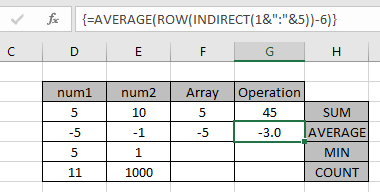The array formula works fine with the AVERAGE function to return value as -3.0 .

Reverse Order : array

For the Third array we will use the below formula using the Generic formula explained above. Here the end number is fixed to 1. So the array will be n to 1 .

Use the Formula:

= ABS ROW ( INDIRECT ( E5 & ":" & D5 ) ) - ( D5 + 1 ) )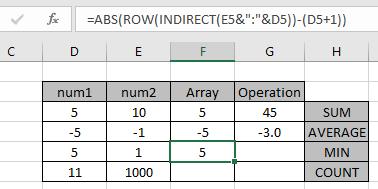Explanation :

After the formula is used in the cell, the single output of the cell is shown as 5 in the gif below. But the array lays inside the cell. When I clicked the formula in the formula bar, and press F9 the cell shows me the array = {5 ; 4 ; 3 ; 2 ; 1 } , the resulting array.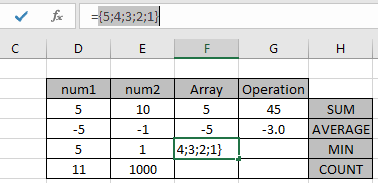After Using the F9 shortcut the array look like as shown in the image above.
Operation: Find the MINIMUM value out the array of numbers from 5 to 1.

For this we will use the MIN function with array formula.

Use the formula in the G3 cell.

{ = MIN ( ABS ( ( ROW ( INDIRECT ( E5 & ":" & D5 ) ) - ( D5 + 1 ) ) )

Explanation :

The above finds the MINIMUM value out the array {5 ; 4 ; 3 ; 2 ; 1 } = 1 .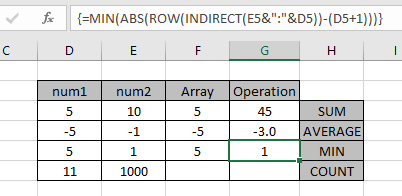Explanation :

The above finds the MINIMUM value out the array {5 ; 4 ; 3 ; 2 ; 1 } = 1 .
The array formula works fine with the MIN function to return value as 1.

Hope you learned how to Create an array of numbers in Excel. Explore more conditional formulas in excel here. You can perform Conditional Formatting in Excel 2016, 2013 and 2010. If you have any unresolved query regarding this article, please do mention below. We will help you.

Related Articles:

How to use the Countif function in excel

Expanding References in Excel

Relative and Absolute Reference in Excel

Shortcut To Toggle Between Absolute and Relative References in Excel

Dynamic Worksheet Reference

All About Named Ranges In Excel

Total number of rows in range in excel

Dynamic Named Ranges in Excel

Popular Articles

50 Excel Shortcut to Increase Your Productivity

Edit a dropdown list

Absolute reference in Excel

If with conditional formatting

If with wildcards

Vlookup by date

Join first and last name in excel

Terms and Conditions of use

The applications/code on this site are distributed as is and without warranties or liability. In no event shall the owner of the copyrights, or the authors of the applications/code be liable for any loss of profit, any problems or any damage resulting from the use or evaluation of the applications/code.